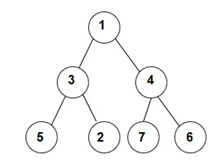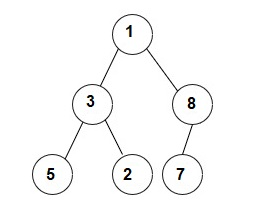# Tree Traversals

Posted: 16 Dec, 2020
Difficulty: Easy

## PROBLEM STATEMENT

#### You have been given a Binary Tree of 'N' nodes, where the nodes have integer values. Your task is to find the ln-Order, Pre-Order, and Post-Order traversals of the given binary tree.

##### For example :
``````For the given binary tree:
````````````The Inorder traversal will be [5, 3, 2, 1, 7, 4, 6].
The Preorder traversal will be [1, 3, 5, 2, 4, 7, 6].
The Postorder traversal will be [5, 2, 3, 7, 6, 4, 1].
``````
##### Input Format :
``````The first line contains an integer 'T' which denotes the number of test cases.

The first line of each test case contains elements of the tree in the level order form. The line consists of values of nodes separated by a single space. In case a node is null, we take -1 in its place.
``````
##### Example :
``````The input for the tree is depicted in the below image:
````````````1 3 8 5 2 7 -1 -1 -1 -1 -1 -1 -1
``````

#### Explanation :

``````Level 1 :
The root node of the tree is 1

Level 2 :
Left child of 1 = 3
Right child of 1 = 8

Level 3 :
Left child of 3 = 5
Right child of 3 = 2
Left child of 8 =7
Right child of 8 =  null (-1)

Level 4 :
Left child of 5 = null (-1)
Right child of 5 = null (-1)
Left child of 2 = null (-1)
Right child of 2 = null (-1)
Left child of 7 = null (-1)
Right child of 7 = null (-1)

1
3 8
5 2 7 -1
-1 -1 -1 -1 -1 -1
``````
##### Note :
``````1. The first not-null node(of the previous level) is treated as the parent of the first two nodes of the current level. The second not-null node (of the previous level) is treated as the parent node for the next two nodes of the current level and so on.

2. The input ends when all nodes at the last level are null(-1).

3. The above format was just to provide clarity on how the input is formed for a given tree. The sequence will be put together in a single line separated by a single space. Hence, for the above-depicted tree, the input will be given as:

1 3 8 5 2 7 -1 -1 -1 -1 -1 -1 -1
``````
##### Output Format :
``````For each test case, return a vector/list of vector/list containing all three traversals (In-Order, Pre-Order, and Post-Order) in each vector/list in the same order.

The first line of output of each test case prints 'N' single space-separated integers denoting the node's values in In-Order traversal.

The second line of output of each test case prints 'N' single space-separated integers denoting the node's values in Pre-Order traversal.

The third and the last line of output of each test case prints 'N' single space-separated integers denoting the node's values in Post-Order traversal.
``````
##### Note :
``````You don't need to print anything, it has already been taken care of. Just implement the given function.
``````
##### Constraints :
``````1 <= T <= 100
0 <= N <= 3000
0 <= data <= 10^9

Where 'data' denotes the node value of the binary tree nodes.

Time limit: 1 sec
``````Approach 1

The idea here is to use recursion for ln-Order, Pre-Order, and Post-Order traversal of a binary tree.

Steps :

Inorder traversal :

void inOrder(‘NODE’):

1. Visit the left subtree of ‘NODE’ i.e., call inOrder(‘NODE’ -> left).
2. Visit node and if ‘NODE’ != NULL then add data of node to answer.
3. Visit the right subtree of ‘NODE’ i.e, call inOrder(‘NODE’ -> right).

Preorder traversal :

void preOrder(‘NODE’):

1. Visit node and if ‘NODE’ != NULL then add data of node to answer.
2. Visit the left subtree of ‘NODE’ i.e., call preOrder(‘NODE’ -> left).
3. Visit the right subtree of ‘NODE’ i.e., call preOrder(‘NODE’ -> right).

Postorder traversal :

void postOrder(‘NODE’):

1. Visit the left subtree of ‘NODE’ i.e., call postOrder(‘NODE’->left).
2. Visit the right subtree of node i.e., call postOrder(‘NODE’->right).
3. Visit node and if ‘NODE’ != NULL then add data of node to answer.